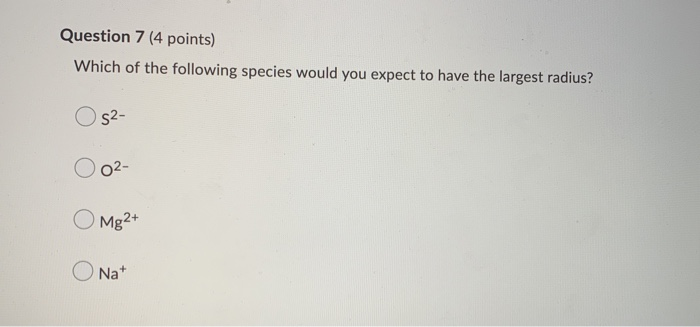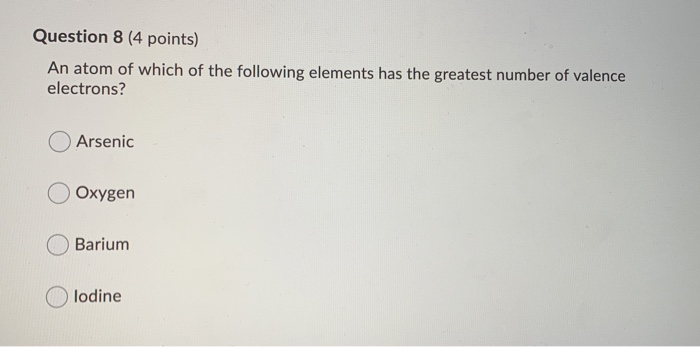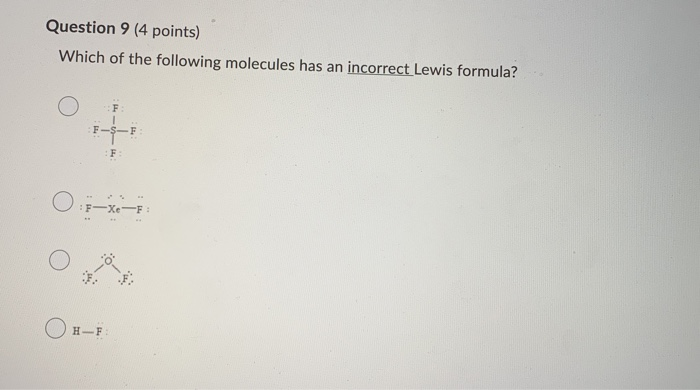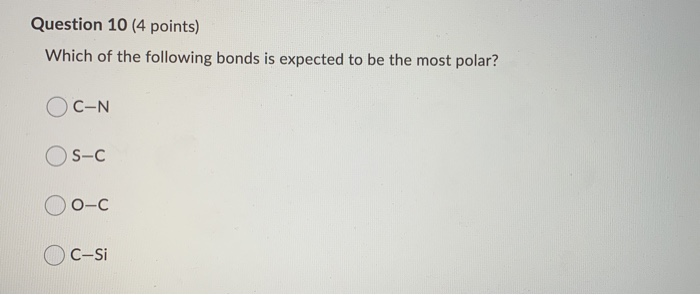# Lewis dot structure for mgsWhich of the following is the Lewis dot structure for one formula unit of magnesium sulfide? me si Question 7 (4 points) Which of the following species would you expect to have the largest radius? Os2- O 0²- O Mg2+ O Nat Question 8 (4 points) An atom of which of the following elements has the greatest number of valence electrons? Arsenic Oxygen Barium Olodine Question 9 (4 points) Which of the following molecules has an incorrect Lewis formula? Ο F Οτ-κι- Ο Ο Question 10 (4 points) Which of the following bonds is expected to be the most polar? OC-N OS-C O o-c Oc-si

6. The Lewis Structure of Magnesium Sulfide is :

The 2nd Option

7. The Correct Option is :

S​​​​​​2-

8. The Correct Option is :

Iodine

​​​​​​​9. The Correct Option is the First
Option :

SF​​​​​4

10. The Correct Option is :

​​​​​​​O-C